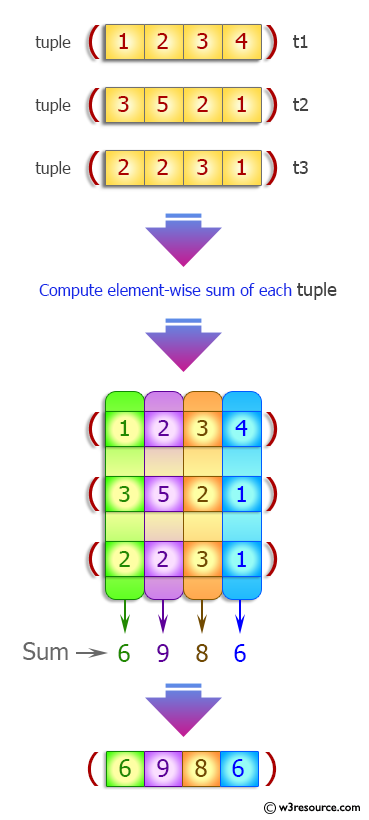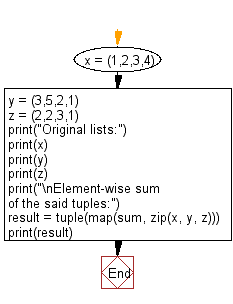﻿ Python: Compute element-wise sum of given tuples - w3resource# Python Exercise: Compute element-wise sum of given tuples

## Python tuple: Exercise-31 with Solution

Write a Python program to compute element-wise sum of given tuples.

Sample Solution:-

Python Code:

``````x = (1,2,3,4)
y = (3,5,2,1)
z = (2,2,3,1)
print("Original lists:")
print(x)
print(y)
print(z)
print("\nElement-wise sum of the said tuples:")
result = tuple(map(sum, zip(x, y, z)))
print(result)
```
```

Sample Output:

```Original lists:
(1, 2, 3, 4)
(3, 5, 2, 1)
(2, 2, 3, 1)

Element-wise sum of the said tuples:
(6, 9, 8, 6)
```

Pictorial Presentation:Flowchart:## Visualize Python code execution:

The following tool visualize what the computer is doing step-by-step as it executes the said program:

Python Code Editor:

Have another way to solve this solution? Contribute your code (and comments) through Disqus.

What is the difficulty level of this exercise?

Test your Programming skills with w3resource's quiz.

﻿

## Python: Tips of the Day

Decapitalizes the first letter of a string:

Example:

```def tips_decapitalize(s, upper_rest=False):
return s[:1].lower() + (s[1:].upper() if upper_rest else s[1:])
print(tips_decapitalize('PythonTips'))
print(tips_decapitalize('PythonTips', True))
```

Output:

```pythonTips
pYTHONTIPS
```